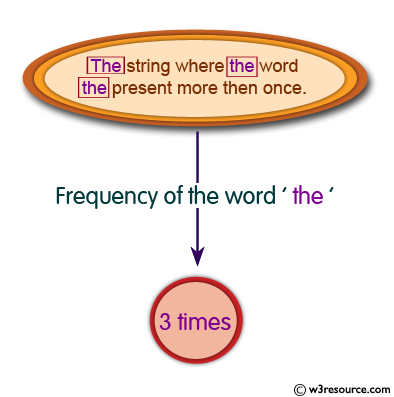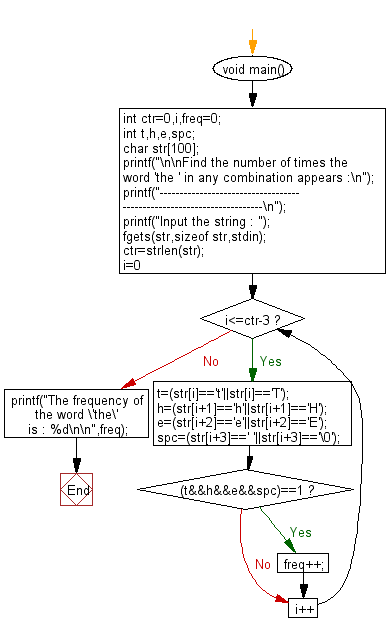﻿ C exercises: Find the number of times the word "the" in any combination appears - w3resource# C Programming: Find the number of times the word 'the' in any combination appears

## C String: Exercise-16 with Solution

Write a program in C to find the number of times a given word 'the' appears in the given string.Sample Solution:

C Code:

``````#include <stdio.h>
#include <string.h>
void main()
{
int ctr=0,i,freq=0;
int t,h,e,spc;
char str;

printf("\n\nFind the number of times the word 'the ' in any combination appears :\n");
printf("----------------------------------------------------------------------\n");

printf("Input the string : ");
fgets(str,sizeof str,stdin);

ctr=strlen(str);

/*Counts the frequency of the word 'the' with a trailing space*/
for(i=0;i<=ctr-3;i++)
{
t=(str[i]=='t'||str[i]=='T');
h=(str[i+1]=='h'||str[i+1]=='H');
e=(str[i+2]=='e'||str[i+2]=='E');
spc=(str[i+3]==' '||str[i+3]=='\0');
if ((t&&h&&e&&spc)==1)
freq++;
}
printf("The frequency of the word \'the\' is : %d\n\n",freq);
}
```
```

Sample Output:

```Find the number of times the word 'the ' in any combination appears :
----------------------------------------------------------------------
Input the string : The stering where the word the present more then onces.
The frequency of the word 'the' is : 3
```

Flowchart :C Programming Code Editor:

Improve this sample solution and post your code through Disqus.

What is the difficulty level of this exercise?

﻿

## C Programming: Tips of the Day

Is there a way to specify how many characters of a string to print out using printf()?

The basic way is:

`printf ("Here are the first 8 chars: %.8s\n", "A string that is more than 8 chars"); `

The other, often more useful, way is:

`printf ("Here are the first %d chars: %.*s\n", 8, 8, "A string that is more than 8 chars"); `

Here, you specify the length as an int argument to printf(), which treats the '*' in the format as a request to get the length from an argument.

You can also use the notation:

```printf ("Here are the first 8 chars: %*.*s\n",
8, 8, "A string that is more than 8 chars"); ```

This is also analogous to the "%8.8s" notation, but again allows you to specify the minimum and maximum lengths at runtime - more realistically in a scenario like:

`printf("Data: %*.*s Other info: %d\n", minlen, maxlen, string, info); `

The POSIX specification for printf() defines these mechanisms.

Ref: https://bit.ly/395Zj2j